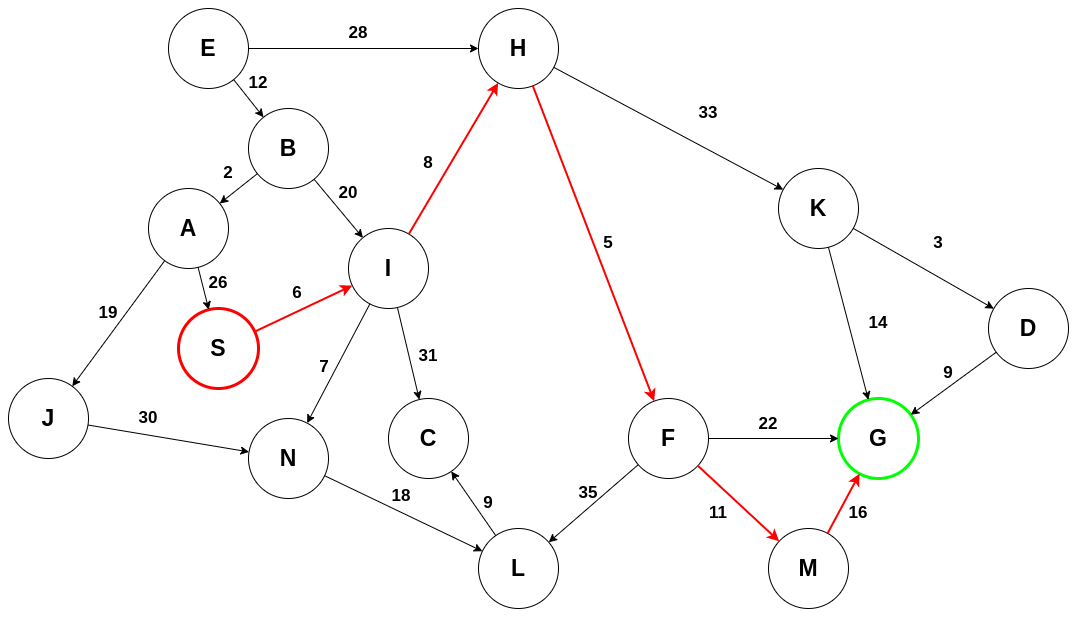# BEAM Search

BEAM Search, a variant of breadth-first Search, is an algorithm that searches a weighted graph for an optimal path from some start node S to some goal node G. The difference between BEAM search and breadth-first search is that at every level of the search tree, only the top β candidates are chosen for further exploration. Here, β is known as the beam width. The reasoning behind this is that a path from S to G is likely to pass through some top number of most promising nodes. This leads to an algorithm that is fast and memory-efficient because it can disregard many nodes that may appear to be too far from the goal. This, however, sometimes causes the algorithm to overlook a potential shortest path because a candidate node is greater than the beam width. This renders the algorithm as incomplete, meaning that finding the shortest path is not guaranteed. Since the algorithm can find a reasonably short path, trading completeness for efficiency is accepted. Much like A* search and best-first search, an evaluation function is used to evaluate the candidacy of nodes for further exploration.

## The Algorithm

Similar to A* search, the algorithm uses an open list and a closed list for the exploration of nodes. In this algorithm, the open list is an ordinary queue. The algorithm begins with a start node S, which also serves as the root node for the search tree.

1. Initialize a search tree with the root node being the start node S. This is level zero in the tree.
2. Add S to the closed list and evaluate all nodes adjacent to S.
3. Select the top β nodes and add them as child nodes to S in the tree. Disregard all other nodes.
4. Add the selected nodes to the open list for further exploration.
5. Remove all nodes in the open list. Once a node is removed from the open list for exploration, add it to the closed list.
6. Evaluate all adjacent nodes and sort them according to the evaluation function.
7. Select the top β nodes and add them to the tree, connecting them to their respective parent nodes.
8. Repeat this process until the goal node G is removed from the open list, indicating that a path has been found.

It is important to note that every level of the search tree contains at most β number of nodes.

## Example

Consider the following example of searching a graph from S to G. For simplicity, only the edge weights will be used for evaluation. A beam width of β = 2 will be used, meaning only the top two nodes are considered at every level.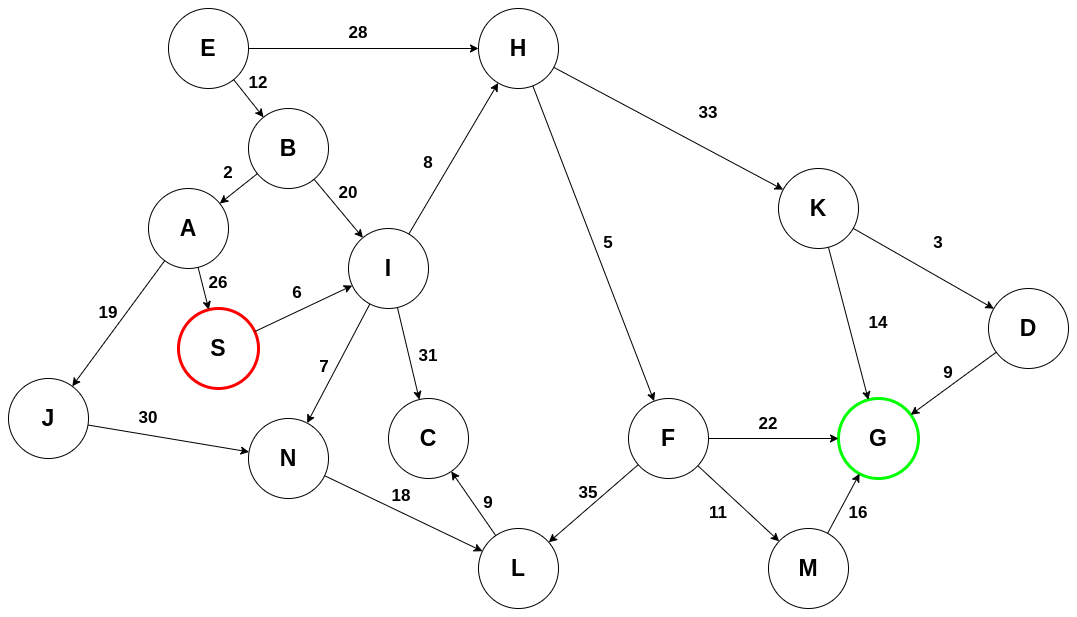Initialize the tree and add S to the closed list: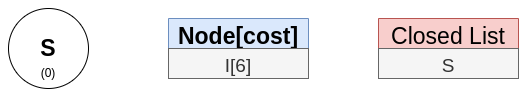S only has one adjacent node, so it is added to the tree: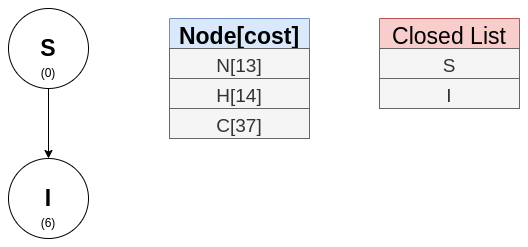Explore I. Nodes N and H are the top two nodes, so those are added to the tree: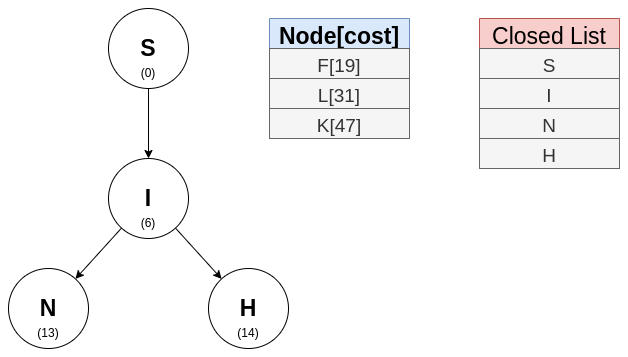Nodes F (adjacent to H in the graph) and L (adjacent to N in the graph) are next: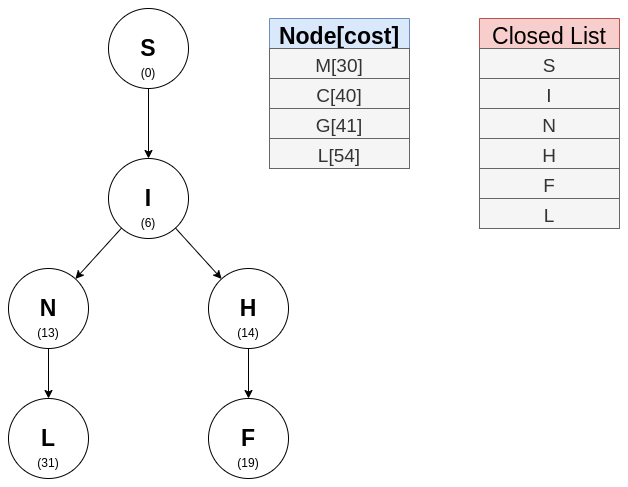The incompleteness of this algorithm can be seen in this step. Node G has appeared in the open list but unfortunately, it has been eliminated by the beam width. Nodes M and C are next: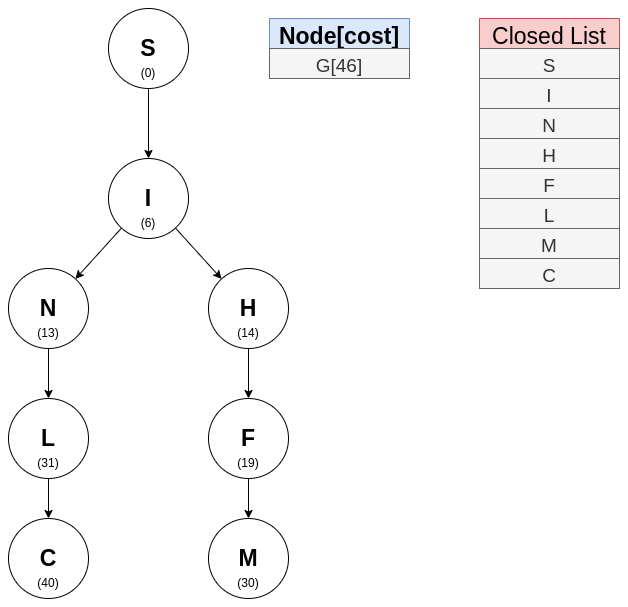It is in this step that node G has been found: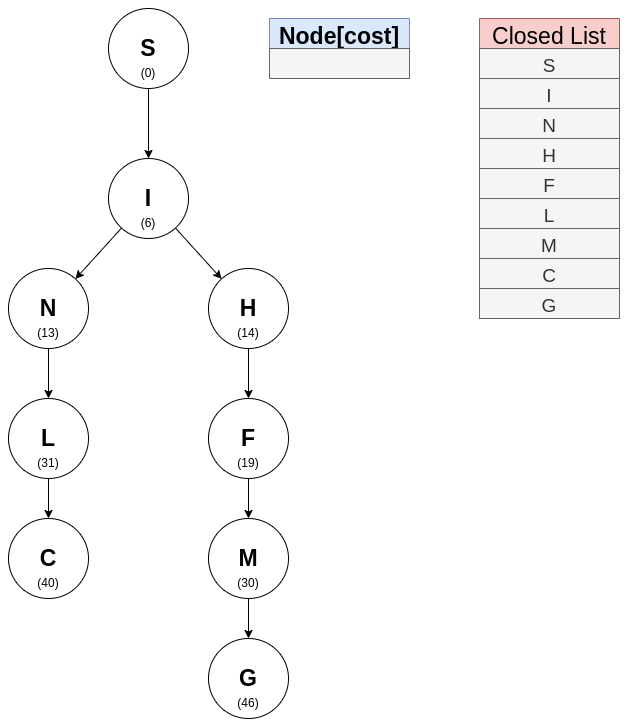Trace the path from S to G: